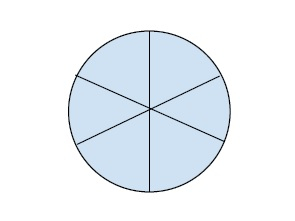# Position of a person diametrically opposite on a circle in C++

In this problem, we are given two integers N and M. there is a circle and N people are standing on it. M denotes the position of a person. Our task is to print the position of the person opposite to M.

Let’s take an example to understand the problem,

Input − N = 6, M = 3

Output − 6

ExplanationTo solve this problem, there will be two cases, one if the position is greater than half the position (second half), the opposition will be the first half and vise versa.

Let’s create a formula for this mathematically,

Case 1 − if m > n/2, the position of the opposite person is m - (n/2)

Case 2 − if m =< n/2, the position of the opposite person is m + (n/2)

## Example

Program to show the illustration of our solution,

Live Demo

#include <iostream>
using namespace std;
void printOppositePosition(int n, int m) {
int pos;
if (m > (n / 2))
pos = (m - (n / 2));
else
pos = (m + (n / 2));
cout<<pos;
}
int main() {
int N = 8, M = 4;
cout<<"The position of person opposite to person at position "<<M<<" when "<<N<<" people are standing in a circle is ";
printOppositePosition(N, M);
return 0;
}

## Output

The position of person opposite to person at position 4 when 8 people are standing in a circle is 8